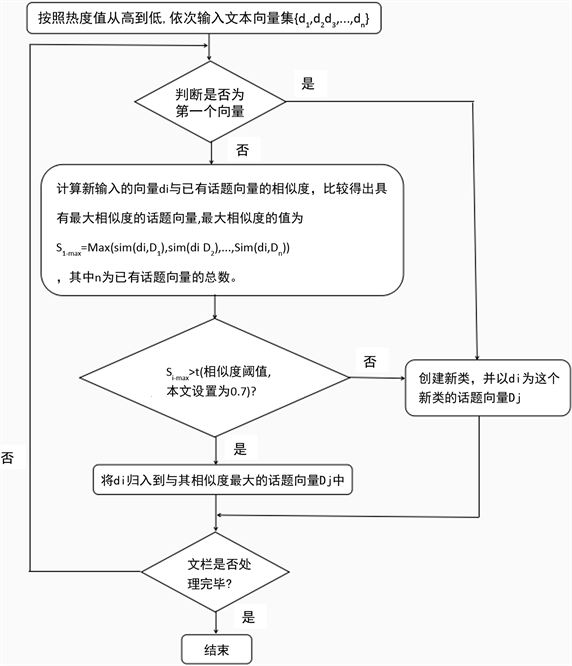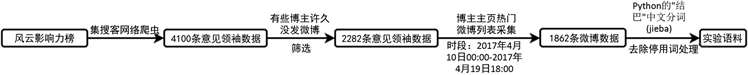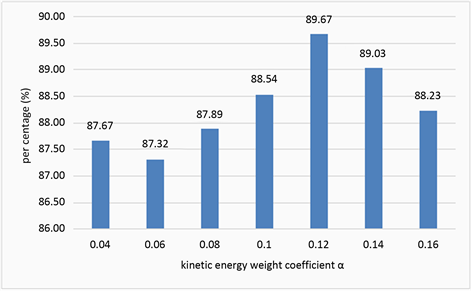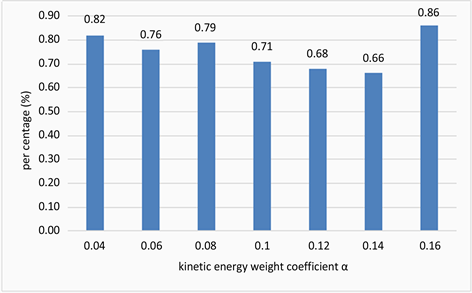# 基于词语动能聚类的社会化媒体热点话题发现建模与优化方法 Modeling and Optimization of Hot Topic Discovery in Social Media Based on Clustering of Word Kinetic Energy

• 全文下载: PDF(2210KB)    PP.40-51   DOI: 10.12677/ECL.2019.81006
• 下载量: 271  浏览量: 886   国家自然科学基金支持

In the context of social media, social media public opinion has become a new perspective of social public opinion. Social big data analytics is showing an increasingly important social and business value. In the emerging field of social big data management, the discovery of hot topics in microblog is the basic and important issues for government public opinion analysis and data management; people have been studying and exploring advanced and applicable theories and methods of hot topic mining. However, when the traditional clustering algorithm is used in the microblog topic detection, the eigenvectors are too sparse and over-dimensioned, resulting in inaccurate clustering results. Therefore, this article explores the sudden features of words in the cycle of topic communication, and proposes the Word Kinetic Energy Clustering (WKEC) model and algorithm. The text clustering model, based on the topic life cycle feature, introduces the concept of kinetic energy theorem in physics, and calculates the kinetic energy of words with the maximum growth rate in the explosion period, which will be added to the weight of the words, modifying the classic TF-IDF model. Based on the algorithm design of Single-Pass and the real dataset from Sina Microblogs, the experimental results show that WKEC model can enhance the text features and improve the accuracy of topic discovery. In addition, due to the strong real-time of microblogging topic, in order to get closer to the real microblogging hot topic list, this article introduces the attenuation coefficient into the calculation of topic heat, and takes the tail time point of the explosion period as the decay moment of topic heat, proposing a more realistic method for the calculation of topic heat.

1. 引言

2. 研究现状

3. 新的热点话题发现方法与建模的理论基础

3.1. 考虑时变因素的话题热度(人气度)的计算

$\begin{array}{l}In{f}_{b,u}\left(t\right)=-d{\left(t-{t}_{0}\right)}^{2}+|{u}_{\text{followed}}|\\ {H}_{T}=\sum \Delta In{f}_{T}=\sum \left(\underset{i=1}{\overset{n}{\sum }}In{f}_{{b}_{i}}\right)\end{array}$ (1)

3.2. 词语动能

“词语动能”(Word Kinetic Energy, WKE)的定义：词语因为运动而具有的能量，称为“词语动能”。在微博话题传播的情境中，“词语运动”是指词语随着文本数量增长而增长的这样一个动态过程，根据词语的增长速度计算而得到的一种值，即“词语动能”。词语的动能，是网络词语的突发性大小的一种度量，用词语的平均增长速度来进行计算 。词语动能的物理意义表示词语所具有的能量，是一种对词语突发性的衡量；而词语动能的社会意义，则表现为话题传播影响力的强度。

$E=\left(1/2\right)m{\nu }^{2}$ (2)

${v}_{k,j}=\left\{\begin{array}{l}0,k=0\\ \frac{{m}_{k,j}-{m}_{k-1,j}}{{T}_{k-1,k}},k\ne 0\end{array}$ (3)

${T}_{k-1,k}={T}_{{k}_{-}middle}-{T}_{k-{1}_{-}middle}$ (4)

${E}_{k,j}=\alpha \cdot t{f}_{kj}\cdot {v}_{k,j}^{2}$ (5)

4. 问题描述与模型构建

4.1. 问题定义

1) 研究问题一：如何基于传播周期对词语突发性进行衡量与评估？

2) 研究问题二：如何基于传播周期对话题热度(人气度)进行时效性考量？

4.2. 基于词语动能的文本聚类模型

1) 对经典特征词权重计算方法TF-IDF的改进

${\omega }_{ij}=\frac{t{f}_{ij}\cdot \mathrm{log}\left(N/{n}_{j}+0.01\right)}{\sqrt{{{\sum }_{p=1}^{k}\left[t{f}_{ij}\cdot \mathrm{log}\left(N/{n}_{j}+0.01\right)\right]}^{2}}}+{E}_{k,j}$ (6)

2) 引入词语动能的文本相似度的计算

$\mathrm{cos}\left(x,y\right)=\frac{{\sum }_{k=1}^{n}{\omega }_{xk}×{\omega }_{yk}}{\sqrt{{\sum }_{k=1}^{n}{\omega }_{xk}^{2}×{\sum }_{k=1}^{n}{\omega }_{yk}^{2}}}$ (7)

4.3. 优化算法设计Figure 1. Flow chart of Single-Pass clustering algorithm

4.4. 基于传播周期的话题热度(人气度)计算

$T{H}_{i}={\sum }_{k=0}^{n}b{h}_{ik}-\beta {\left(t-{t}_{0}\right)}^{2}={\sum }_{k=0}^{n}\left(2r{e}_{k}+c{m}_{k}+\sqrt{f{l}_{k}}\right)-\beta {\left(t-{t}_{o}\right)}^{2}$ (8)

5. 实验及结果分析

5.1. 实验语料准备Figure 2. Flow chart for acquiring experimental corpus

5.2. 模型和算法性能评测

TDT会议对话题检测制定了一种规范的评价标准，评测指标主要有：召回率、准确率、漏检率、错检率、F值以及误测开销 。该评价标准同样适用于微博平台的话题检测。本文选用话题检测常用的评价指标即召回率、错检率来对模型与算法的性能进行测试。

$R=D/T\ast 100%$ (9)

${P}_{FA}=FA/NT\ast 100%$ (10)

5.3. 实验结果

5.3.1. 动能权重系数 $\alpha$ 的取值Figure 3. Recall rate changing with $\alpha$Figure 4. Error detection rate changing with $\alpha$

5.3.2. 实验结果及算法评测Table 1. Experimental results of topic discovery algorithmTable 2. Comparison of experimental resultsTable 3. Topic recall rate obtained by two algorithmsTable 4. Topic error detection rate obtained by two algorithms

6. 结论

  Lee, I. (2017) Big Data: Dimensions, Evolution, Impacts, and Challenges. Business Horizons, 60, 293-303. https://doi.org/10.1016/j.bushor.2017.01.004  冯芷艳, 郭迅华, 曾大军, 等. 大数据背景下商务管理研究若干前沿课题[J]. 管理科学学报, 2013, 16(1): 1-9.  Ahmad, S.N. and Laroche, M. (2017) Analyzing Electronic Word-of-Mouth: A Social Commerce Constructs. International Journal of Information Management, 37, 202-213. https://doi.org/10.1016/j.ijinfomgt.2016.08.004  郑大庆, 黄丽华, 张成洪, 等. 大数据治理的概念及其参考架构[J]. 研究与发展管理, 2017, 29(4): 65-72.  刘社瑞, 唐双. 自媒体时代微博舆情演化与应对策略[J]. 求索, 2011(10): 86-87, 171.  杜治娟. 社会媒体大数据分析研究综述[J]. 计算机科学与探索, 2017, 11(1): 1-23.  唐晓波, 房小可. 基于文本聚类与LDA相融合的微博主题检索模型研究[J]. 情报理论与实践, 2013, 36(8): 85-90.  徐雅斌, 李卓, 吕非非, 等. 基于频繁词集聚类的微博新话题快速发现[J]. 系统工程理论与实践, 2014, 34(S1): 276-282.  梁晓贺, 田儒雅, 吴蕾, 等. 基于超网络的微博舆情主题挖掘方法[J]. 情报理论与实践, 2017, 40(10): 100-105.  黄发良, 冯时, 王大玲, 等. 基于多特征融合的微博主题情感挖掘[J]. 计算机学报, 2017, 40(4): 872-888.  Yan, X. and Zhao, H. (2013) Chinese Microblog Topic Detection Based on the Latent Semantic Analysis and Structural Property. Journal of Networks, 8, 917-923. https://doi.org/10.4304/jnw.8.4.917-923  Yang, L., Lin, H.F., Lin, Y., et al. (2016) Detection and Extraction of Hot Topics on Chinese Microblogs. Cognitive Computation, 8, 577-586. https://doi.org/10.1007/s12559-015-9380-6  Li, W.J., Feng, Y.M., Li, D.J., et al. (2016) Micro-Blog Topic Detection Method Based on BTM Topic Model and K-Means Clustering Algorithm. Automatic Control and Computer Sciences, 50, 271-277. https://doi.org/10.3103/S0146411616040040  Zhao, Y.Y., Qin, B., Liu, T., et al. (2016) Social sentiment Sensor: A Visualization System for Topic Detection and Topic Sentiment Analysis on Microblog. Multimedia Tools and Applications, 75, 8843-8860. https://doi.org/10.1007/s11042-014-2184-y  袁华, 徐华林, 钱宇, 等. 域内海量数据中热点话题及其特征词抽取方法[J]. 管理工程学报, 2018, 32(4): 133-140.  牛奉高, 张亚宇. 基于共现潜在语义向量空间模型的语义核构建[J]. 情报学报, 2017, 36(8): 834-842.  薛薇. 基于突发性诊断的网络热点事件识别方法[J]. 统计与决策, 2015(15): 8-12.  仲兆满, 管燕, 李存华, 等. 微博网络地域Top-K突发事件检测[J]. 计算机学报, 2018, 41(7): 1504-1516.  郑斐然, 苗夺谦, 张志飞, 等. 一种中文微博新闻话题检测的方法[J]. 计算机科学, 2012, 39(1): 138-141.  林思娟, 林柏钢, 许为, 等. 一种基于词语能量值变化的微博热点话题发现方法研究[J]. 信息网络安全, 2015(10): 46-52.  金镇晟. 基于改进的TF-IDF算法的中文微博话题检测与研究[D]: [硕士学位论文]. 北京: 北京理工大学, 2015: 26-29.  Wu, Y. and Wu, W. (2015) Modeling Topic Popularity Dis-tribution and Evolution in an Online Discussion Forum. Journal of Computational Information Systems, 18, 6797-6810.  何跃, 蔡博驰. 基于因子分析法的微博热度评价模型[J]. 统计与决策, 2016(18): 52-54.  裴可锋, 陈永洲, 马静. 基于DTPM模型的话题热度预测方法[J]. 情报杂志, 2016, 35(12): 52-57.  杨冠超. 微博客热点话题发现策略研究[D]: [硕士学位论文]. 杭州: 浙江大学, 2011: 26-27.  薛峰, 周亚东, 高峰, 等. 一种突发性热点话题在线发现与跟踪方法[J]. 西安交通大学学报, 2011, 45(12): 64-69 + 116.  姚海波. 微博热点话题检测与趋势预测研究[D]: [硕士学位论文]. 广州: 华南理工大学, 2013: 23-38.  孙胜平. 中文微博客热点话题检测与跟踪技术研究[D]: [硕士学位论文]. 北京: 北京交通大学, 2011: 38-40.  洪宇, 张宇, 刘挺, 等. 话题检测与跟踪的评测及研究综述[J]. 中文信息学报, 2007(6): 71-87.  孟小峰, 李勇, 祝建华. 社会计算: 大数据时代的机遇与挑战[J]. 计算机研究与发展, 2013(12): 2483-2491.  吴应良, 黄媛, 王选飞. 在线中文用户评论研究综述: 基于情感计算的视角[J]. 情报科学, 2017(6): 159-163, 170.  王玮, 温世阳. 情感分析在社会化媒体效果研究中的应用——基于分类序列规则的微博文本情绪分析[J]. 国际新闻界, 2017, 39(4): 63-75.